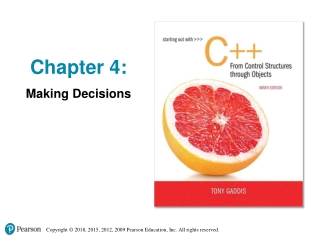# Chapter 4: Making Decisions - PowerPoint PPT PresentationDownload PresentationChapter 4: Making Decisions

Download Presentation## Chapter 4: Making Decisions

- - - - - - - - - - - - - - - - - - - - - - - - - - - E N D - - - - - - - - - - - - - - - - - - - - - - - - - - -
##### Presentation Transcript

1. Chapter 4: Making Decisions

2. 4.1 Relational Operators

3. Relational Operators • Used to compare numbers to determine relative order • Operators:

4. Relational Expressions • Boolean expressions – true or false • Examples: 12 > 5 is true 7 <= 5 is false if x is 10, then x == 10 is true, x != 8 is true, and x == 8 is false

5. Relational Expressions • Can be assigned to a variable: result = x <= y; • Assigns 0 for false, 1 for true • Do not confuse = and ==

6. 4.2 The if Statement

7. The if Statement • Allows statements to be conditionally executed or skipped over • Models the way we mentally evaluate situations: • "If it is raining, take an umbrella." • "If it is cold outside, wear a coat."

8. Flowchart for Evaluating a Decision

9. Flowchart for Evaluating a Decision

10. The if Statement • General Format: if (expression) statement;

11. The if Statement-What Happens To evaluate: if (expression) statement; • If the expression is true, then statement is executed. • If the expressionis false, then statement is skipped.

12. if Statement in Program 4-2 Continued…

13. if Statement in Program 4-2

14. Flowchart for Program 4-2 Lines 21 and 22

15. if Statement Notes • Do not place ; after (expression) • Place statement; on a separate line after (expression), indented: if (score > 90) grade = 'A'; • Be careful testing floats and doubles for equality • 0 is false; any other value is true

16. 4.3 Expanding the if Statement

17. Expanding the if Statement • To execute more than one statement as part of an if statement, enclose them in { }: if (score > 90) { grade = 'A'; cout << "Good Job!\n"; } • { } creates a block of code

18. 4.4 The if/else Statement

19. The if/else statement • Provides two possible paths of execution • Performs one statement or block if the expression is true, otherwise performs another statement or block.

20. The if/else statement • General Format: if (expression) statement1; // or block else statement2; // or block

21. if/else-What Happens To evaluate: if (expression) statement1; else statement2; • If the expression is true, then statement1 is executed and statement2 is skipped. • If the expression is false, then statement1 is skipped and statement2 is executed.

22. The if/else statement and Modulus Operator in Program 4-8

23. Flowchart for Program 4-8 Lines 14 through 18

24. Testing the Divisor in Program 4-9 Continued…

25. Testing the Divisor in Program 4-9

26. 4.5 Nested if Statements

27. Nested if Statements • An if statement that is nested inside another if statement • Nested if statements can be used to test more than one condition

28. Flowchart for a Nested if Statement

29. Nested if Statements • From Program 4-10

30. Nested if Statements • Another example, from Program 4-1

31. Use Proper Indentation!

32. 4.6 The if/else ifStatement

33. The if/else if Statement • Tests a series of conditions until one is found to be true • Often simpler than using nested if/else statements • Can be used to model thought processes such as:"If it is raining, take an umbrella, else, if it is windy, take a hat, else, take sunglasses”

34. if/else if Format if (expression) statement1; // or block else if (expression) statement2; // or block . . // other else ifs . else if (expression) statementn; // or block

35. The if/else if Statement in Program 4-13

36. Using a Trailing else to Catch Errors in Program 4-14 • The trailing else clause is optional, but it is best used to catch errors. This trailing else catches invalid test scores

37. 4.7 Flags

38. Flags • Variable that signals a condition • Usually implemented as a bool variable • Can also be an integer • The value 0 is considered false • Any nonzero value is considered true • As with other variables in functions, must be assigned an initial value before it is used

39. 4.8 Logical Operators

40. Logical Operators • Used to create relational expressions from other relational expressions • Operators, meaning, and explanation:

41. Logical Operators-Examples int x = 12, y = 5, z = -4;

42. The logical && operator in Program 4-15

43. The logical || Operator in Program 4-16

44. The logical ! Operator in Program 4-17

45. Logical Operator-Notes • ! has highest precedence, followed by &&, then || • If the value of an expression can be determined by evaluating just the sub-expression on left side of a logical operator, then the sub-expression on the right side will not be evaluated (short circuit evaluation)

46. 4.9 Checking Numeric Ranges with Logical Operators

47. Checking Numeric Ranges with Logical Operators • Used to test to see if a value falls inside a range: if (grade >= 0 && grade <= 100) cout << "Valid grade"; • Can also test to see if value falls outside of range: if (grade <= 0 || grade >= 100) cout << "Invalid grade"; • Cannot use mathematical notation: if (0 <= grade <= 100) //doesn’t work!